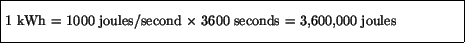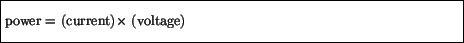Next: Magnetism and magnetic fields Up: Charges in motion Previous: Resistance

## Electrical Power

In our every day lives we use electrical energy to do work for us. This electrical energy is provided either by our power company through the outlets in our wall, or we can buy stored electrical energy in the form of batteries. Different electrical appliances use electrical energy at different rates. As described in Sub-section 4.2, power is the rate at which work is done. The power rating of an appliance tells us the rate at which it uses electrical energy. For example, a 1500 watt hair dryer uses 1500 joules of electrical energy per second. The power companies charge us for the total amount of energy we use during a billing period. Most bills quote electrical energy consumption in kilowatt hours (kWh's). One kilowatt hour is defined as the amount of energy used by a one kilowatt appliance (i.e. one that uses 1000 joules per second) that is run for one hour. If you know the power rating of an appliance you can easily calculate how much it will cost you to run. For example, keeping a 60 watt light bulb on for a full day uses 60 watts x 24 hours = 1440 watt hours = 1.44 kilowatt hours of energy. At 9 cents per kilowatt hour this would cost just under 15 cents. Since kilowatt hour is a unit of energy, we can convert it to joules. We need to multiply the power by the time, soThus, if you pay approximately 9 cents for each kilowatt hour that you use, ten cents pays for almost four million joules of energy. If power companies quoted their rates in terms of joules, the costs would seem much more reasonable!

In any electrical appliance their is a simple relationship between the power output of a component, the potential difference across that component, and the current running through it. This is because charge times potential difference is energy, so (charge per unit time) times (potential difference) gives (energy per unit time). Thus, we have that:For example, in a nine volt battery, a current of 1 Ampère means that nine joules per second (i.e. 9 watts) of energy are being used. Conversely, if you are using a 60 watt light bulb plugged into a 110 volt wall socket, the current running through it is 60 watts/ 110 volts, or about half an Ampére. A 120 watt light bulb will run at twice that current.Next: Magnetism and magnetic fields Up: Charges in motion Previous: Resistance
modtech@theory.uwinnipeg.ca
1999-09-29